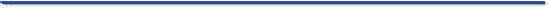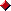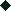The JavaTM TutorialTrail: Learning the Java Language
Lesson: Language Basics

## Arithmetic Operators

The Java programming language supports various arithmetic operators for all floating-point and integer numbers. These operators are `+` (addition), `-` (subtraction), `*` (multiplication), `/` (division), and `%` (modulo). The following table summarizes the binary arithmetic operations in the Java programming language.

Binary Arithmetic Operators
Operator Use Description
`+` `op1 + op2` Adds `op1` and `op2`; also used to concatenate strings
`-` `op1 - op2 ` Subtracts `op2` from `op1`
`*` `op1 * op2` Multiplies `op1` by `op2`
`/` `op1 / op2` Divides `op1` by `op2`
`%` `op1 % op2` Computes the remainder of dividing `op1` by `op2`

Here's an example program, `ArithmeticDemo`, that defines two integers and two double-precision floating-point numbers and uses the five arithmetic operators to perform different arithmetic operations. This program also uses `+` to concatenate strings. The arithmetic operations are shown in boldface:

```public class ArithmeticDemo {
public static void main(String[] args) {

//a few numbers
int i = 37;
int j = 42;
double x = 27.475;
double y = 7.22;
System.out.println("Variable values...");
System.out.println("    i = " + i);
System.out.println("    j = " + j);
System.out.println("    x = " + x);
System.out.println("    y = " + y);

System.out.println("    i + j = " + (i + j));
System.out.println("    x + y = " + (x + y));

//subtracting numbers
System.out.println("Subtracting...");
System.out.println("    i - j = " + (i - j));
System.out.println("    x - y = " + (x - y));

//multiplying numbers
System.out.println("Multiplying...");
System.out.println("    i * j = " + (i * j));
System.out.println("    x * y = " + (x * y));

//dividing numbers
System.out.println("Dividing...");
System.out.println("    i / j = " + (i / j));
System.out.println("    x / y = " + (x / y));

//computing the remainder resulting from dividing numbers
System.out.println("Computing the remainder...");
System.out.println("    i % j = " + (i % j));
System.out.println("    x % y = " + (x % y));

//mixing types
System.out.println("Mixing types...");
System.out.println("    j + y = " + (j + y));
System.out.println("    i * x = " + (i * x));
}
}
```
The output from this program is:
```Variable values...
i = 37
j = 42
x = 27.475
y = 7.22
i + j = 79
x + y = 34.695
Subtracting...
i - j = -5
x - y = 20.255
Multiplying...
i * j = 1554
x * y = 198.37
Dividing...
i / j = 0
x / y = 3.8054
Computing the remainder...
i % j = 37
x % y = 5.815
Mixing types...
j + y = 49.22
i * x = 1016.58
```
Note that when an integer and a floating-point number are used as operands to a single arithmetic operation, the result is floating point. The integer is implicitly converted to a floating-point number before the operation takes place. The following table summarizes the data type returned by the arithmetic operators, based on the data type of the operands. The necessary conversions take place before the operation is performed.

Result Types of Arithmetic Operations
Data Type of Result Data Type of Operands
`long` Neither operand is a `float` or a `double` (integer arithmetic); at least one operand is a `long`.
`int` Neither operand is a `float` or a `double` (integer arithmetic); neither operand is a `long`.
`double` At least one operand is a `double`.
`float` At least one operand is a `float`; neither operand is a `double`.

In addition to the binary forms of `+` and `-`, each of these operators has unary versions that perform the following operations, as shown in the next table:

Unary Arithmetic Operators
Operator Use Description
`+` `+op` Promotes `op` to `int` if it's a `byte`, `short`, or `char`
`-` `-op` Arithmetically negates `op`

Two shortcut arithmetic operators are `++`, which increments its operand by 1, and `--`, which decrements its operand by 1. Either `++` or `--` can appear before (prefix) or after (postfix) its operand. The prefix version, `++op`/`--op`, evaluates to the value of the operand after the increment/decrement operation. The postfix version, `op++`/`op--`, evaluates to the value of the operand before the increment/decrement operation.

The following program, called `SortDemo`, uses `++` twice and `--` once.

```public class SortDemo {
public static void main(String[] args) {
int[] arrayOfInts = { 32, 87, 3, 589, 12, 1076,
2000, 8, 622, 127 };

for (int i = arrayOfInts.length; --i >= 0; ) {
for (int j = 0; j < i; j++) {
if (arrayOfInts[j] > arrayOfInts[j+1]) {
int temp = arrayOfInts[j];
arrayOfInts[j] = arrayOfInts[j+1];
arrayOfInts[j+1] = temp;
}
}
}

for (int i = 0; i < arrayOfInts.length; i++) {
System.out.print(arrayOfInts[i] + " ");
}
System.out.println();
}
}
```
This program puts ten integer values into an array — a fixed-length structure that can hold multiple values of the same type — then sorts them. The boldface line of code declares an array referred to by `arrayOfInts`, creates the array, and puts ten integer values into it. The program uses `arrayOfInts.length` to get the number of elements in the array. Individual elements are accessed with this notation: `arrayOfInts[index]`, where `index` is an integer indicating the position of the element within the array. Note that indices begin at 0. You’ll get more details and examples for arrays in the section Arrays.

The output from this program is a list of numbers sorted from lowest to highest:

```
3 8 12 32 87 127 589 622 1076 2000
```
Let's look at how the `SortDemo` program uses `--` to help control the outer of its two nested sorting loops. Here's the statement that controls the outer loop:
```for (int i = arrayOfInts.length; --i >= 0; ) {
...
}
```
The `for` statement is a looping construct, which you'll meet later in this chapter. What's important here is the code in boldface, which continues the `for` loop as long as the value returned by `--i` is greater than or equal to 0. Using the prefix version of `--` means that the last iteration of this loop occurs when `i` is equal to 0. If we change the code to use the postfix version of `--`, the last iteration of this loop occurs when `i` is equal to `-1`, which is incorrect for this program because `i` is used as an array index and `-1` is not a valid array index.

The other two loops in the program use the postfix version of `++`. In both cases, the version used doesn't really matter, because the value returned by the operator isn't used for anything. When the return value of one of these shortcut operations isn't used for anything, convention prefers the postfix version.

The shortcut increment/decrement operators are summarized in the following table.

Shortcut Arithmetic Operators
Operator Use Description
`++` `op++` Increments `op` by 1; evaluates to the value of `op` before it was incremented
`++` `++op` Increments `op` by 1; evaluates to the value of `op` after it was incremented
`--` `op--` Decrements `op` by 1; evaluates to the value of `op` before it was decremented
`--` `--op` Decrements `op` by 1; evaluates to the value of `op` after it was decremented